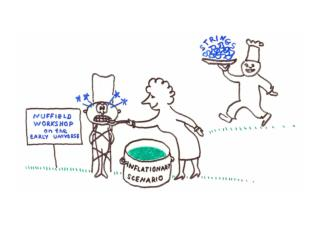Download PresentationMEASURES OF THE MULTIVERSE

# MEASURES OF THE MULTIVERSE

Download Presentation## MEASURES OF THE MULTIVERSE

- - - - - - - - - - - - - - - - - - - - - - - - - - - E N D - - - - - - - - - - - - - - - - - - - - - - - - - - -
##### Presentation Transcript

1. MEASURES OF THE MULTIVERSE Alex Vilenkin Tufts Institute of Cosmology Cambridge, Dec. 2007

2. STRING THEORY PREDICTS MULTIPLE VACUA WITH DIFFERENT CONSTANTS OF NATURE Bousso & Polchinski (2000) Susskind (2003) Douglas (2003) “THE LANDSCAPE” Eternal inflation the entire landscape will be explored.

3. THE MEASURE PROBLEM: What is the probability for a randomly picked observer (“reference object”)to be in a given type of vacuum? Assume we have a model for calculating the numbers of reference objects. Still there is a problem with infinities NOTE: A measure is needed even for predicting the CMB multipoles.

4. PLAN Structure of the multiverse General requirements for the measure Proposalsand problems The noodle measure

5. Spacetime structure i+ i+ Bubbles (pocket universes) ? ? ? Bubbles nucleate and expand at nearly the speed of light. Terminal & recyclable bubbles Eternal geodesics Inflating spacetimes are past-incomplete.

6. What is at the past boundary? Quantum nucleation from nothing Chaotic initial conditions Not relevant for the measure (almost).

7. The measure problem i+ i+ Bubbles (pocket universes) The number of bubbles is infinite, even in a finite comoving volume. The number of ref. objects in each bubble is infinite. Need a cutoff.

8. Global time measures i+ t = const Use a hypersurface t = const as a cutoff. Linde, Linde & Mezhlumian (1994) Garcia-Bellido, Linde & Linde (1994) The limit at does not depend on the initial state. BUT: depends on what we use as t. (Most of the reference objects are near cutoff.)

9. Requirements for the measure Measure axioms: Independence of initial conditions. Independence of time parametrization.

10. The pocket-based measure Garriga, Tanaka & A.V. (1999) Garriga, Schwartz-Perlov, A.V. & Winitzki (2005) Bubble abundance Weight factor (characterizes the number of reference objects per bubble).

11. The bubble abundance: The geodesics project all bubbles onto . Geodesic congruence Include only bubbles of projected volume bigger than . Then let . are independent of the choice of congruence and of . independent of initial conditions: dominated by bubbles formed at late times. An equivalent prescription: Easther, Lim & Martin (2005)

12. Calculation of pj -- fraction of co-moving volume in vacuum of type i Probability per Hubble time to get to vacuum i from vacuum j. Gained from other vacua Lost to other vacua Highest nonvanishing eigenvalue of (it can be shown to be negative). Corresponding eigenvector (it is non-degenerate). for bubbles of type j in parent vacuum if bubble nucleation rate is small Reduces to an eigenvalue problem

13. The weight factor Sample equal comoving volumes in all bubbles. i+ Internal FRW geometry: Bubble spacetimes are identical at small : Sample comoving spheres: Same for all bubbles

14. Bubble nucleation rate Slow-roll expansion inside the bubble Note: large inflation inside bubbles is rewarded.

15. Some other proposals: A version of global time cutoff: Use different cutoff times tjfor different bubbles. A.V. (1994), Linde (2007) Pjare approximatelytime-parameter independent. Include only observer’s past light cone. Bousso (2006) Vanchurin (2007) Pjdepend on the initial state. Volume weighting of histories. Hawking (2006) Hartle, Hawking & Hertog (2007) Divergent; needs a cutoff. Bousso (2007) Violates additivity. The pocket-based measure satisfies all requirements.

16. The main shortcoming of pocket-based measure: Does not account for bubble collisions. Bubbles form infinite clusters; have fractal structure. The cluster-based (“noodle”) measure Garriga, Guth & A.V.

17. The noodle measure All clusters within the same parent vacuum are statistically equivalent. -- probability to be in a bubble of type j in a cluster of type . Calculate cluster abundance with the same prescription as we used for bubbles. assuming low bubble nucleation rate

18. The noodle measure Sample equal comoving spheres in all clusters (?) Spacelike version of time-parameter dependence. Sample a tube-like region around a spacelike geodesic – “the noodle”. Stationary solution: assuming low bubble nucleation rate Agrees with pocket-based measure.

19. What is the probability for us to observe a collision with another bubble (i.e. to be in a collision-affected region)?

20. Reducible landscapes The landscape splits into several sectors inaccessible from one another. Probability of vacuum j in sector A: Probability of j, calculated using the hypersurface Nucleation probability with vacuum k

21. CONCLUSIONS We formulated requirements for the measure: Additivity; independence of initial conditions; independence of time parametrization. The noodle measure is applicable to general bubble spacetimes & satisfies all requirements. Open issues: Uniqueness? Freak observers Extension to quantum diffusion

22. Global time measures i+ t = const Youngness paradox: Most observers evolve at very early cosmic times (high TCMB), when the conditions for life are still hostile. Low probability of evolving is compensated by exponential increase in the volume. The observed (low) value of TCMB is highly unlikely. Linde & Mezhlumian (1996) Guth (2001); Tegmark (2004)